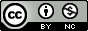Produced in R version 3.6.1.

• Fisher information is a common way to get standard errors in various settings, but is not so suitable for POMP models.

• We often find ourselves working with complex models having some weakly identified parameters for which the asymptotic assumptions behind these standard errors are inadequate.

• Further, the technique requires evaluation of second derivatives of the log likelihood; a numerically unstable problem when one has the capability to obtain only noisy estimates of the log likelihood.

• We suppose that $$\theta\in\mathbb{R}^D$$ and so we can write $$\theta=\theta_{1:D}$$.

• The Hessian matrix of a function is the matrix of its second partial derivatives. We write the Hessian matrix of the log likelihood function as $$\nabla^2\ell(\theta)$$, a $$D\times D$$ matrix whose $$(i,j)$$ element is $\big[\nabla^2\ell(\theta)\big]_{ij} = \frac{\partial^2}{\partial\theta_i\partial\theta_j}\ell(\theta).$

• The observed Fisher information is $I^* = - \nabla^2\ell(\theta^*).$

• A standard asymptotic approximation to the distribution of the MLE for large $$N$$ is $\hat\theta(Y_{1:N}) \approx N[\theta, {I^*}^{-1}],$ where $$\theta$$ is the true parameter value. This asserts that the MLE is asymptotically unbiased, with variance asymptotically attaining the Cramer-Rao lower bound. Thus, we say the MLE is asymptotically efficient.

• A corresponding approximate 95% confidence interval for $$\theta_d$$ is $\theta_d^* \pm 1.96 \big[{I^*}^{-1}\big]_{dd}^{1/2}.$

• We usually only have one time series, with some fixed $$N$$, and so we cannot in practice take $$N\to\infty$$. When our time series model is non-stationary it may not even be clear what it would mean to take $$N\to\infty$$. These asymptotic results should be viewed as nice mathematical reasons to consider computing an MLE, but not a substitute for checking how the MLE behaves for our model and data.

Back